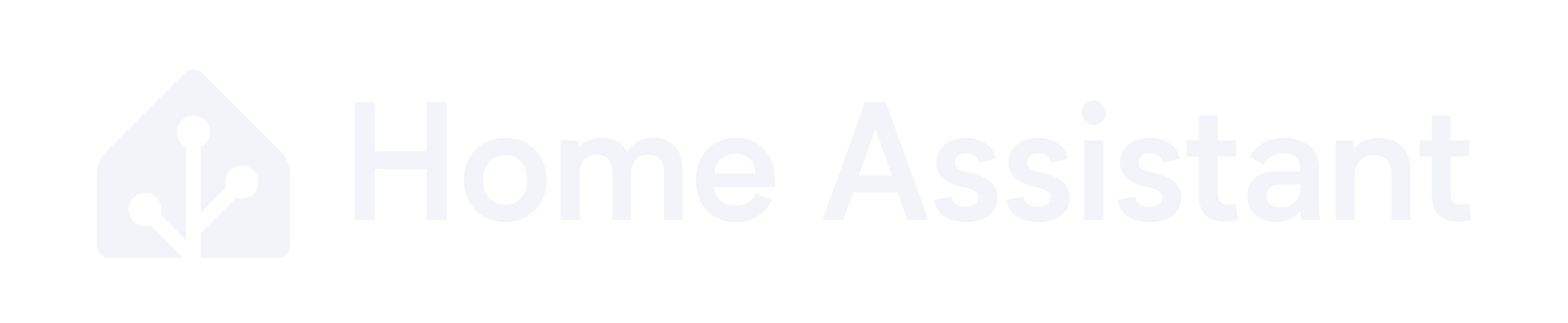# Energy consumption and generation

Hi folks,

Anyone had any experience with using a sensor template that does the following:

1. If sensor value is positive (not negative value) then that should go under Current demand sensor
1a. If the value is negative then it should be 0.

2. If the value if negative then we want that to go to Export sensor but as a positive value (e.g if -4.3 then convert to 4.3)
2a. If value is is positive then it should be 0

Import:

sensor Meter:

• platform: mqtt
state_topic: “raven”
name: “Current Demand”
state_class: “measurement”
device_class: “energy”
unit_of_measurement: ‘kW’
icon: mdi:flash
value_template: >-
{% if “demand” in value_json %}
{{ value_json.demand }}

Export:

sensor Meter2:

• platform: mqtt
state_topic: “raven”
name: “Current Export”
state_class: “measurement”
device_class: “energy”
unit_of_measurement: ‘kW’
icon: mdi:flash
value_template: >-
{% if “demand” in value_json %}
{{ value_json.demand }}
``````{"demand": -4.35, "raw_demand": -4350, "multiplier": 1, "divisor": 1000, "timestamp": "2021-12-01T13:14:53Z"}
``````

name: “Current Demand”

``````value_template: >
{% if "demand" in value_json %}
{{ 0 if value_json.demand <=0 else value_json.demand }}
{% else %}
unknown
{% endif %}
``````

name: “Current Export”

``````value_template: >
{% if "demand" in value_json %}
{{ 0 if value_json.demand > 0 else value_json.demand|abs  }}
{% else %}
unknown
{% endif %}
``````
1 Like

Hey thank you!

That worked perfectly. And thank you for the tip on how to ask for help too.

1 Like

How often is this false?

``````{% if "demand" in value_json %}
``````

Should only be false if there is connectivity issues to the smart meter via zigbee.

Ah ok. If it was a common occurrence you could have set it to the last value, but as it is an uncommon occurrence better to leave it as `unknown` so you know when there is an issue.

1 Like